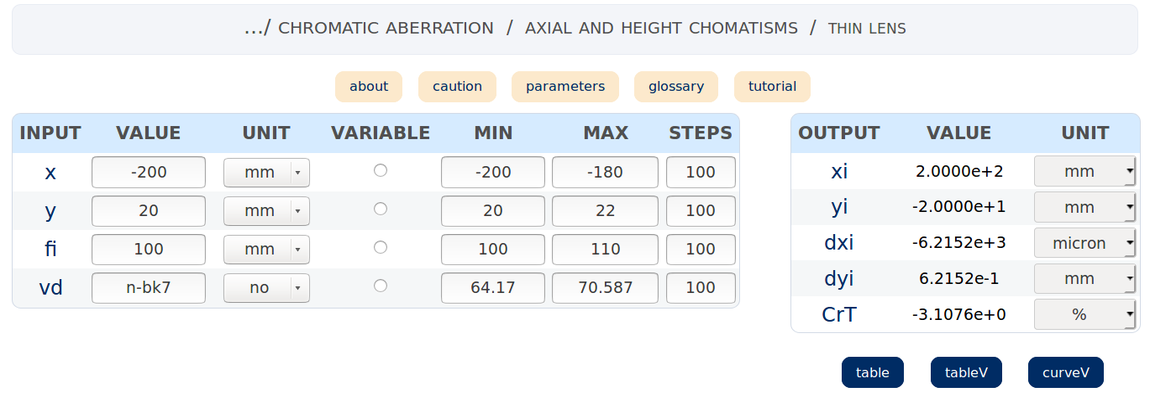This page is optimized for large screens. Zooming may cause some display problems.

Check your OS and browser compatibilty HERE

Handy calculations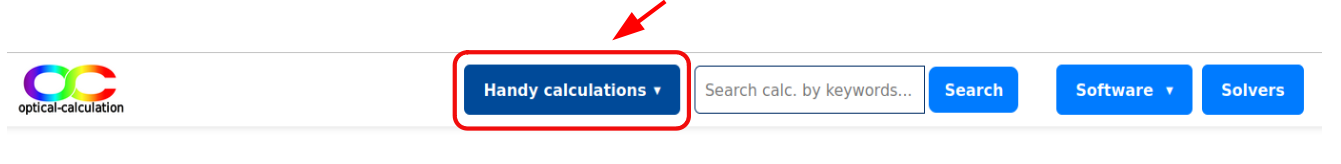The Handy Calculations tool addresses numerous specific calculation cases in various fields of photonics. Indeed, 277 computation pages are proposed in many domains: diffraction, fiber optics, geometric optics, interference, laser, optoelectronics, polarization, radiometry/photometry.

A set of conversions page is also available. The calculation pages integrate a contextual help with tooltips for an improved understanding and a better use.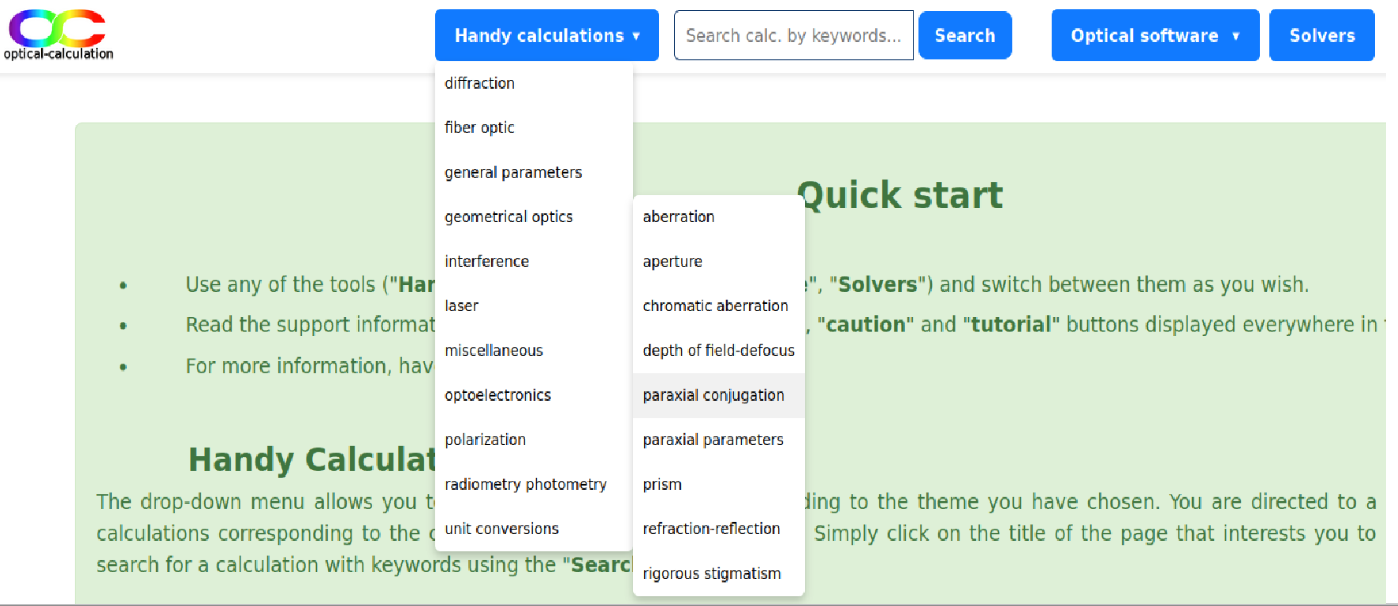The calculation pages are accessible from the drop-down menus displayed after clicking on the "handy calculations" link at the top left of each page. These drop-down menus correspond to the different fields of photonics. The related sub-menus are topics of the considered field.

After clicking on a submenu, a calculation page is displayed by default. The chances are high that it's not the one you want. You can find a calculation page more suited to your needs in the list displayed in the left block of the page (later called "list of links"). If you are not satisfied with any calculation page, please do not hesitate to contact us. We will do our best to answer your request.

Note that the drop-down menus are also displayed in the central block (titled "Handy Calculations") of the home page.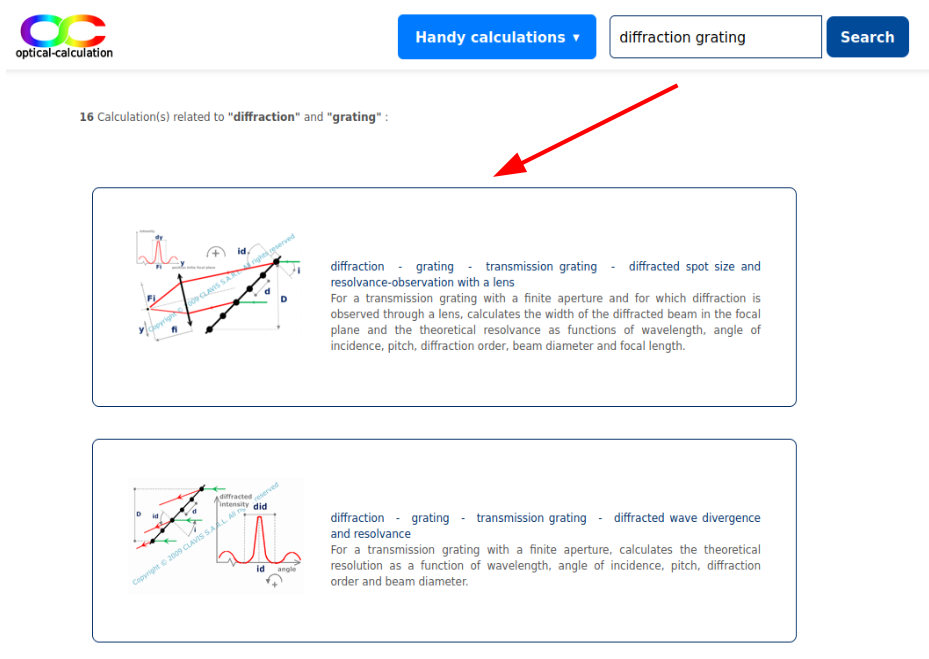An alternative way to find a calculation is to enter keywords in the search cell located in the header. After clicking on the search button, the list of calculations relevant to all key words is displayed. For each relevant calculation, the list includes a title, a short description and eventually a small explanatory scheme. It is possible to access to the calculation by clicking on its title or on its scheme if it exists.

Note that it is also possible to access to a calculation page from the list displayed in the "sitemap" page.

Each calculation page includes several blocks:

- a list of links (3) to calculation pages relevant to the topic (2) (displayed on the left side),

- one or two calculation table(s) (6) depending on the calculation format and the buttons (7) enabling to process the computation,

- link to tooltips and to a tutorial (4) (displayed above the calculation table(s)),

- the path (1) up to the calculation page (displayed above the tooltips and link to a tutorial),

- an explanatory scheme (5) in most of the calculation pages that can be enlarged by clicking on it (at the top left of the page).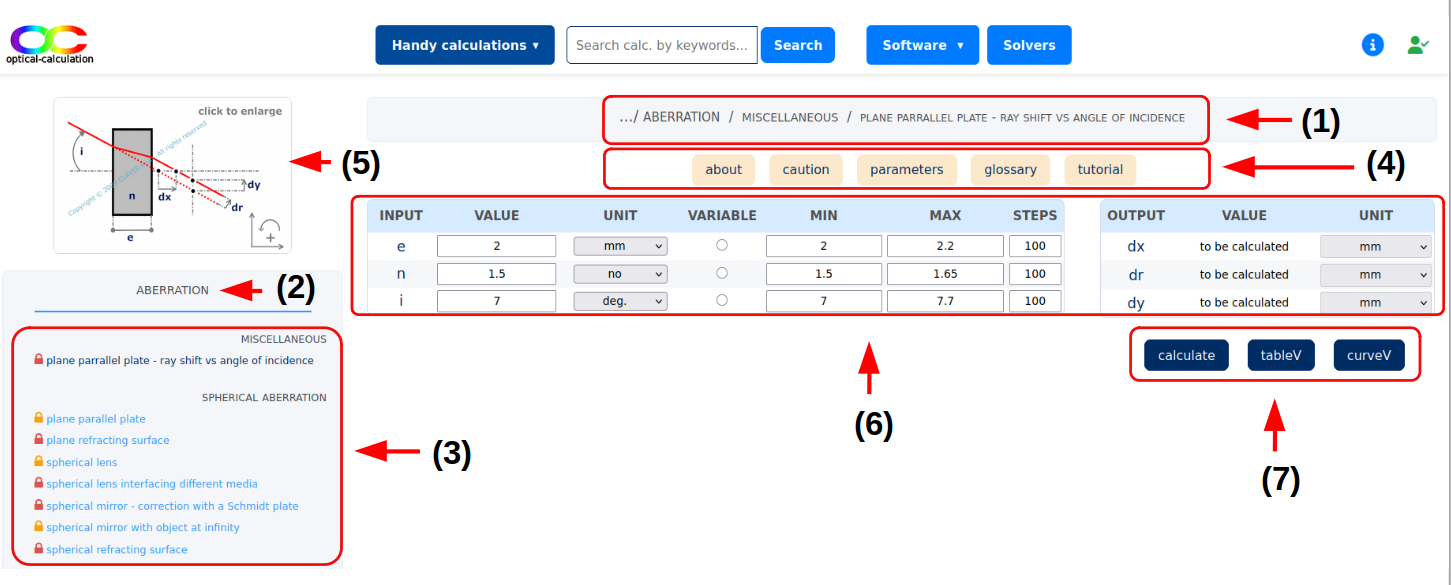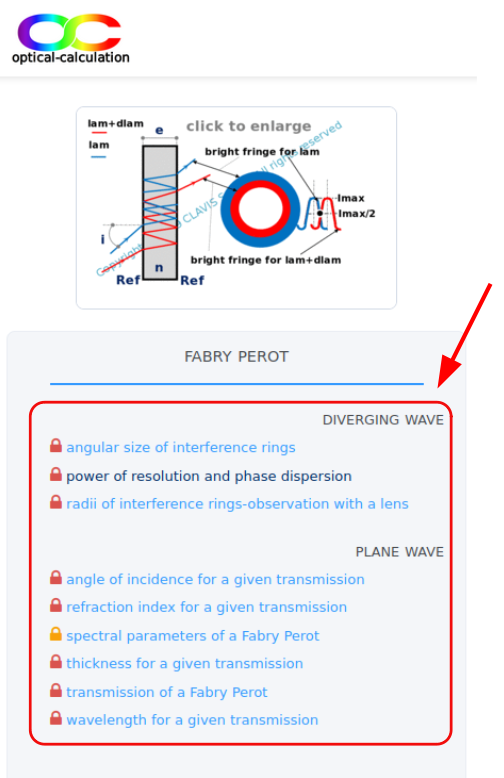By clarity, the list may be organized by sub topics (displayed in grey small caps). The user can access to the calculation of his choice by clicking on its title in the list. the title is then highlighted by appearing with a darker color. A padlock is represented to the left of each calculation title in three different ways:

-   means that the calculation can be processed freely without any connection,
-   means that the calculation can be processed if the user is connected and has a Student or a Premium license,
-   means that the calculation can be processed if the user is connected and has a Premium license only.

More details on the accessibility of the calculations and features are provided in the features page.

Basically, each handy calculation page is used to perform a calculation from input data entered by the user.

There are two calculation modes.

In one mode, called the fixed mode, the calculation is processed with all the input parameters fixed.

In the other mode called the varying mode, one input parameter varies and all other parameters are fixed. The varying mode is possible for all calculation pages except the unit conversion pages, the "glass catalogs" pages and the "wavelengths" page.

Also, there are two possible formats for the calculation pages.

The pages in accordance with the two tables format contain 2 tables, one for entering input parameters ( "input" table) and the other for displaying output parameters ("output" table) in fixed mode.

In the pages in accordance with the single table format, there is only one calculation table ("parameters" table) used for entering the input parameters and display the results in the fixed mode. The user chooses the parameter to calculate by leaving its cell empty. All other parameters are input data. Therefore, each parameter can be calculated from the others.

Depending on the page, the number of buttons for processing a calculation and their label are different. In any case, the buttons for calculating in varying mode are the "tableV" and "curveV" buttons. Obviously, those for calculating in fixed mode are all buttons other than the "tableV" and "curveV" buttons.

Note that the parameters description (input or ouput parameters) is displayed in a tooltip when the mouse hovers over their label.

Calculating in fixed mode

The input/output data units can be selected in the "unit" column. The calculation is realized and the results are displayed in the dedicated table after clicking on the button "calculate" or "table" (one of this button is necessarily displayed on the page). In some pages, the results can also be displayed in a graphic by using the "graphic" or "curve" buttons or any other custom button.

Two tables format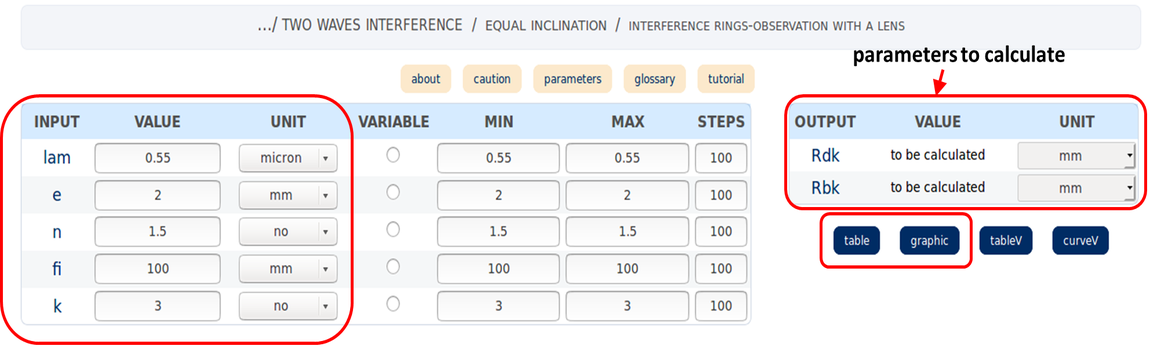Single table format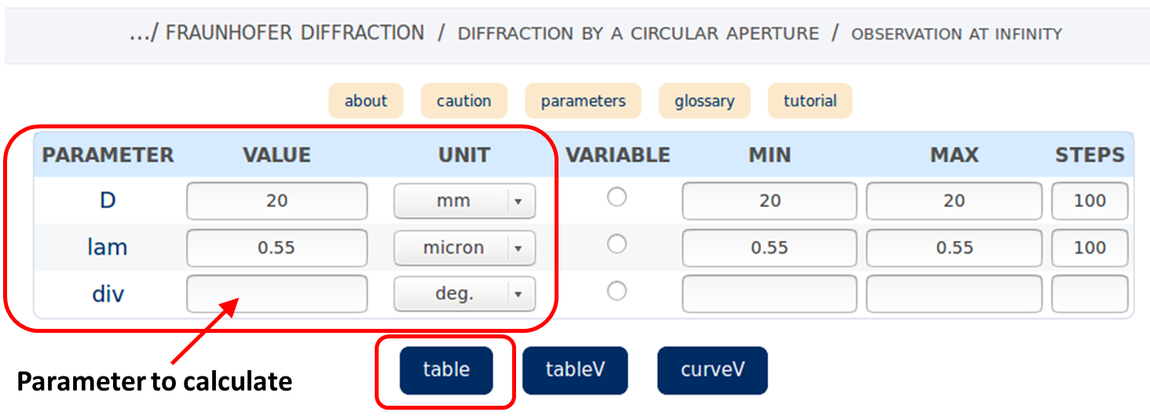The input values can be entered either in numerical or scientific format using a maximum of 14 digits .
The output values are displayed in scientific format with a minimum of 2 decimal places and a maximum of 6 decimal places.

Remember that when using a single table format, the "value" cell corresponding to the parameter to calculate must be cleared otherwise an error message is displayed.

Calculating in varying mode

Except for the conversion pages, it is possible to perform the calculation by varying a parameter in a range of values. The input parameter to be varied is then selected by the corresponding radio button in the "variable" column. Its minimum value valmin and maximum value valmax are entered respectively in the corresponding "MIN" and "MAX" cells. Finally, the number of values +1 to be considered is entered in its "STEPS" cell. The calculations are realized for each value of the parameter: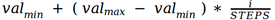.

i varies from "0" to "STEPS".

the input values can be entered either in numerical or scientific format using a maximum of 8 digits. The results are displayed in scientific format. Displayed input and output values use respectively 6 and 4 decimal places. The number of values ("STEPS" columns) is in general limited to 200. However, this maximum value can be decreased for some time-consuming calculations.

Note that, as for the fixed mode, in the single table format, the "value" cell of the parameter to be calculated must necessary be cleared. Also, the variable parameter can obviously not be the one to be calculated.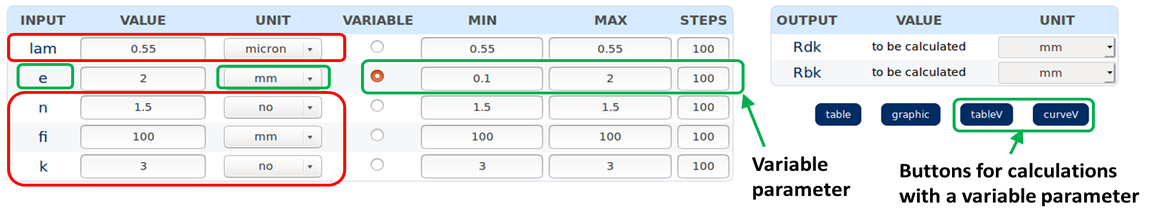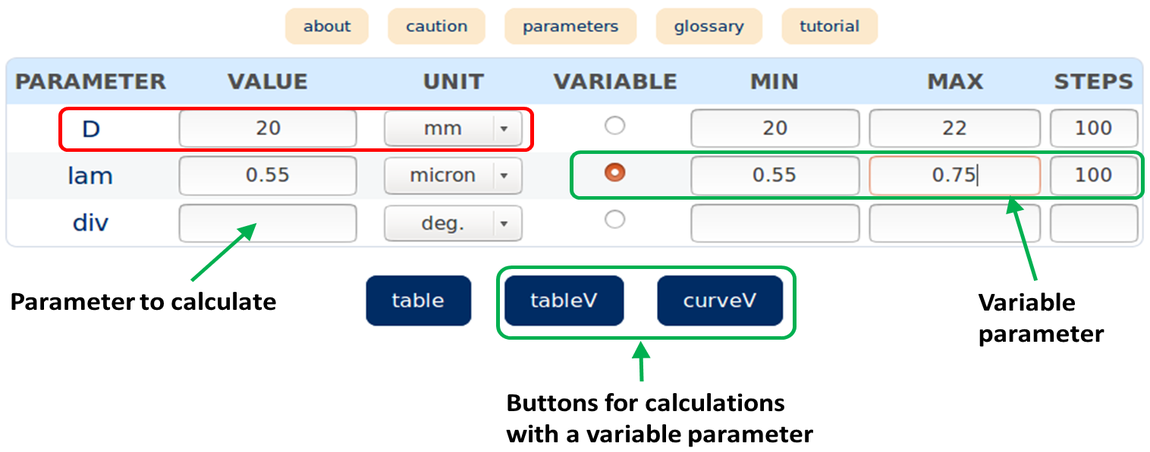The results can be either displayed in a table or in curves.

The results appear in a table after clicking on the "tableV" button. For each output, three columns are displayed. The first column labelled "cod.Er" corresponds to an error code which value is 0 if the calculation has been processed and 1 if it has not been processed because it is not possible or not accurate enough. The second column corresponds to the value of the variable input. The third column is the output value. The fixed input values are diplayed on the top of the table.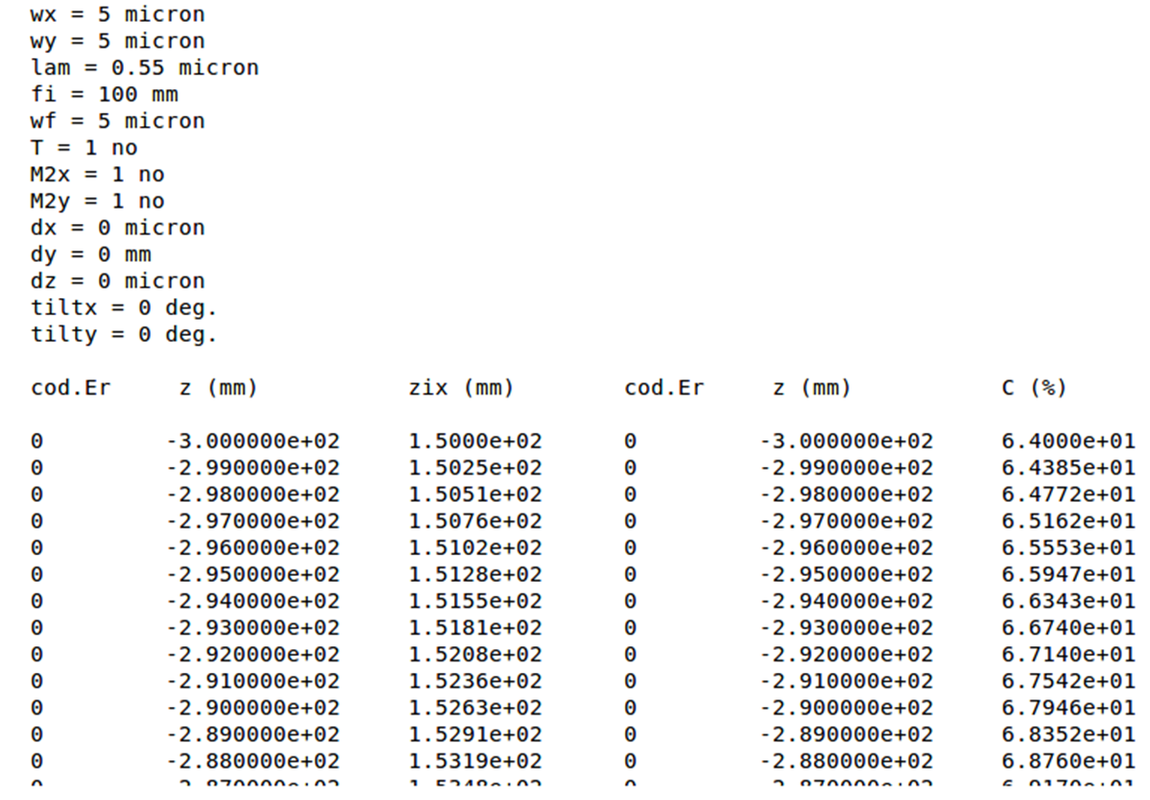The results appear in curves after clicking on the "curveV" button. There are as many curves as output parameters to calculate. For each curve, the abscissa represents the variable parameter values and the ordinate represents the output values. The fixed input values are diplayed on the top of the curve.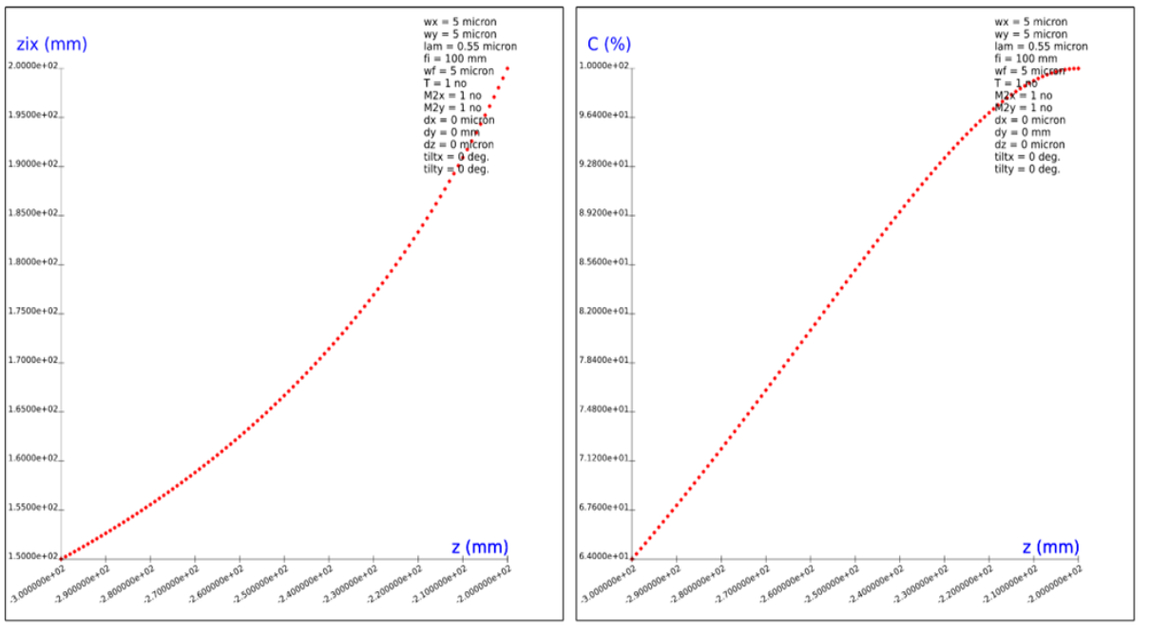Caution

In each page, input cells are filled by default to allow the calculation. These default values are first automatically loaded. In some cases, they lead to an error message because they are not relevant to the computation. This comes from the fact that they may be used in different pages and they cannot necessarily be appropriate to each of the calculations. Next, these default values can be replaced by values entered or computed by the user and can also lead to error messages in certain calculations. One solution would have been to choose different parameters for each calculation page but it was not adopted because it is sometimes useful to use input values or calculated values as input values in other pages without having to re-enter them.

In some pages, the message "not possible" may be displayed in the parameter(s) cell(s) to be calculated without any prior error message. This is because, some intermediate tests are performed during the calculation in order to avoid unrealistically or inaccurate values. Unlike preliminary tests like those preventing from divisions by zero, eventual issues arising from these tests can not be displayed as a full error message and therefore appear directly in the cell(s).

Using very small or very large input values can lead to large rounding errors. In some cases, possible rounding errors are detected. if the calculation is supposed to give a very small or a very large value, then the result will be rounded to a very small or a very large value. The process depends on the calculation but for instance, the results can be rounded to orders of magnitude of 10-6 radians if they are considered as small angle values and 10+12 if they are considered as large dimensions or large distances expressed in mm.

Some mismatchs can happen in calculations using a single table where any parameter can be calculated from all others. There are cases where the display accuracy is not sufficient and computation of an initial input using results from a previous calculation may lead to a different value than the original one. In general, the difference is small. However, there are cases where the difference can be large (for instance, the re-computation of the "dark current" in the "shot noise current" page with very different initial values for the "photocurrent" and "dark current"). Higher display accuracy would solve this issue but would cause other problems in the current display modes.

If the user makes a calculation with a varying parameter, its values will go from a minimum value to a maximum value defined respectively in the related "MIN" and "MAX" cells. These cells are in general filled by default, except for the parameter to be calculated by default in calculation pages using a single table. These minimum and maximum values can be changed at the user convenience in such a way that the maximum value is strictly larger than the minimum one.

Note that for parameters whose default value is zero, the minimum and maximum default values are also both equal to zero and must be changed in order to respect the previous condition.

Note also that those parameters which are integers (like for instance diffraction orders,...) cannot be chosen as variable parameters for the calculations.

Links to tooltips ("about", "caution", "parameters", "glossary") are proposed for a better understanding of the calculation. They can be opened from the menu at the top of the calculation table(s). Here are their functions:

- "about" displays a tooltip explaining what the calculation does.

- "caution" displays a tooltip detailing the main precautions when entering the input data.

- "parameters" displays a tooltip giving the meaning of each parameter involved in the calculation.

- "glossary" displays a tooltip giving the definition of some specific words used in the page or relevant to the calculation.

A link to a "tutorial" related the calculation topic is also displayed on the right of the tooltips menu.

The tooltips appear when the user hover the mouse over its label. It is also possible to display a tooltip content in a separate page by clicking on its label.

Note that in addition to the "parameters" tooltip, the definition of each parameter is also displayed in a pop-up when the user hover the mouse over its label (corresponding to the relevant cell of the "input", "ouput" or "parameters" column).

Here is and example of tooltip displayed when the user hover the mouse on the "caution" link: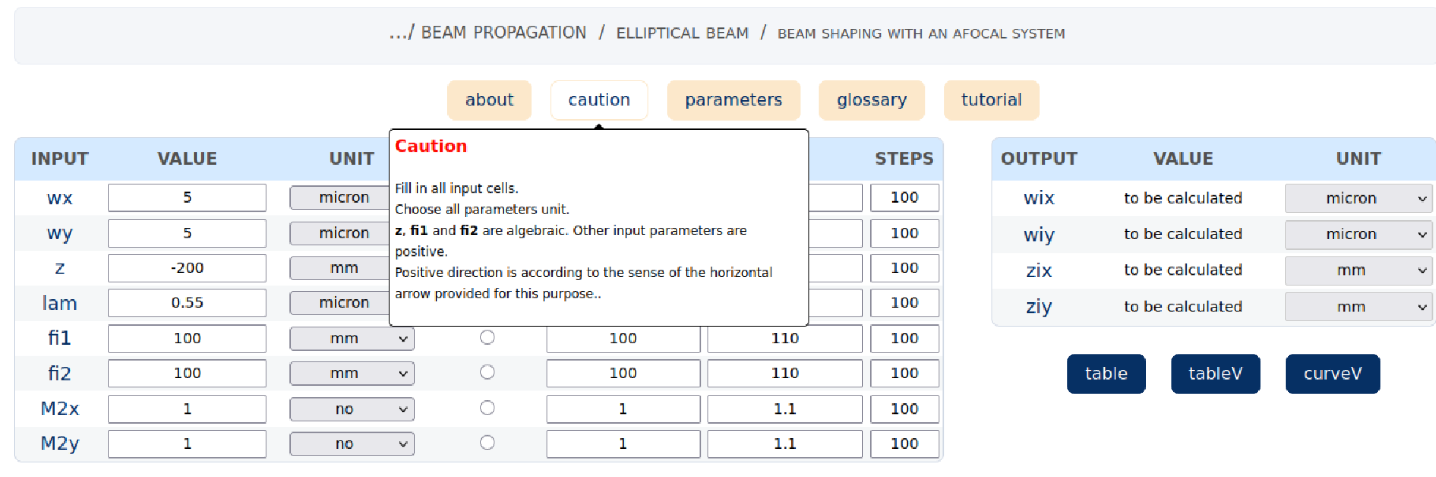Here is an example of tooltip displayed when the user hover the mouse on the "parameters" link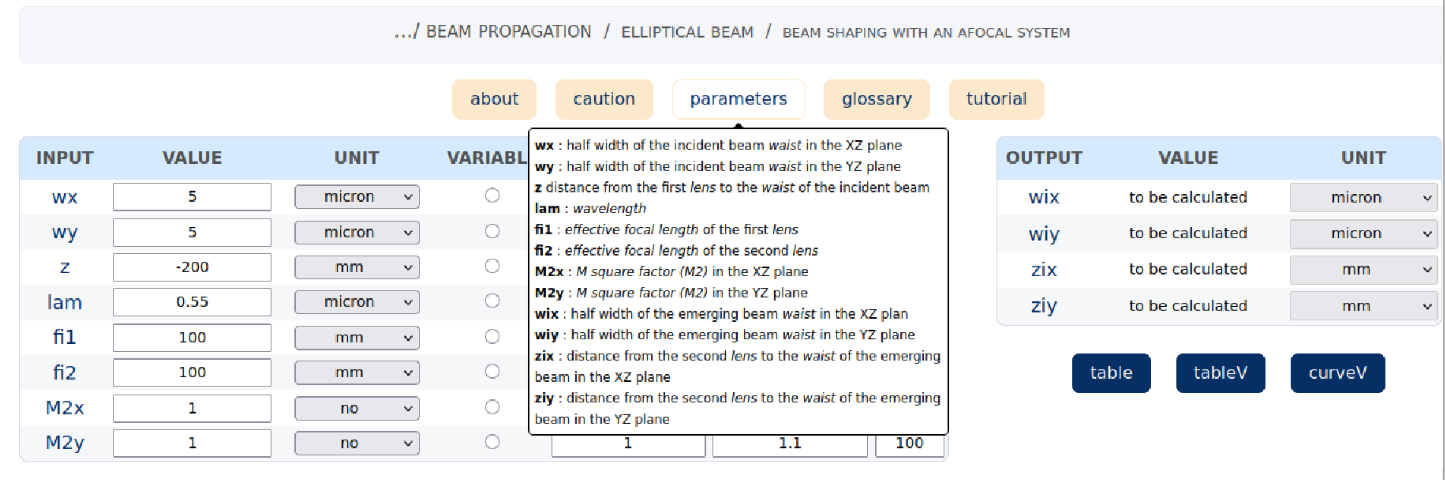Here is an example of pop-up displayed when the user hover the mouse on a parameter label.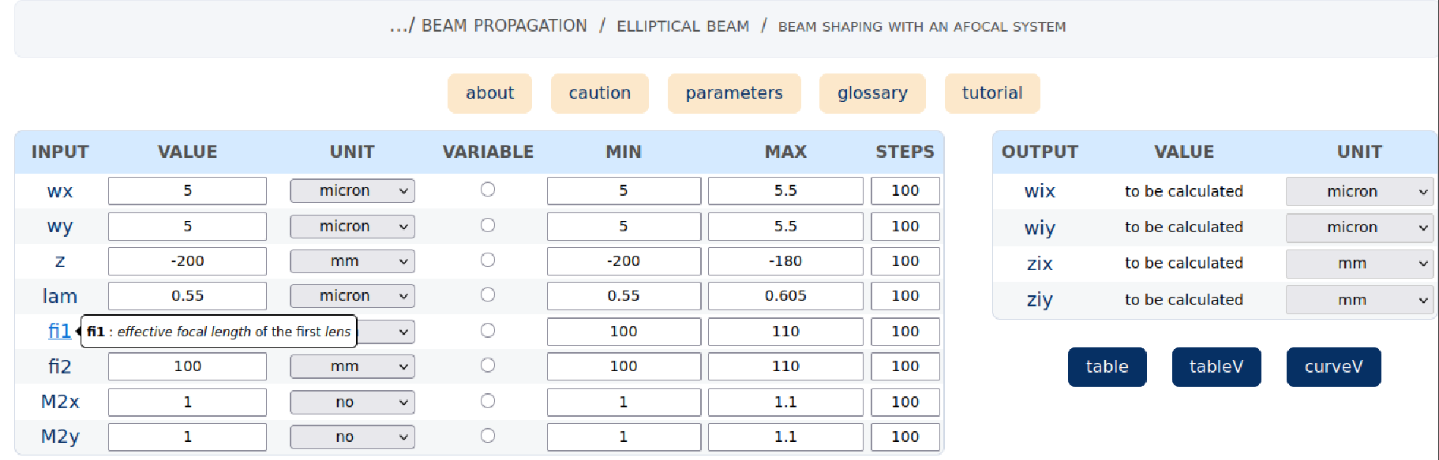In some computation pages, the refraction index is an input parameter. The user can enter either a number or a glass reference

Catalog page

The user can either enter a number or a glass reference from the catalog page. The catalog (path: "handy calculations/General parameters/catalogs").

On the left of this page, several links to catalogs are available. After choosing a catalog, it is possible to select a reference in the "glass" cell" and calculate the refraction index for the wavelength that is entered in the "lam" cell. The constringences and the lowest wavelength insuring an acceptable transmission are also displayed. These values may help to select a reference and check that it is adequate for the calculation of interest.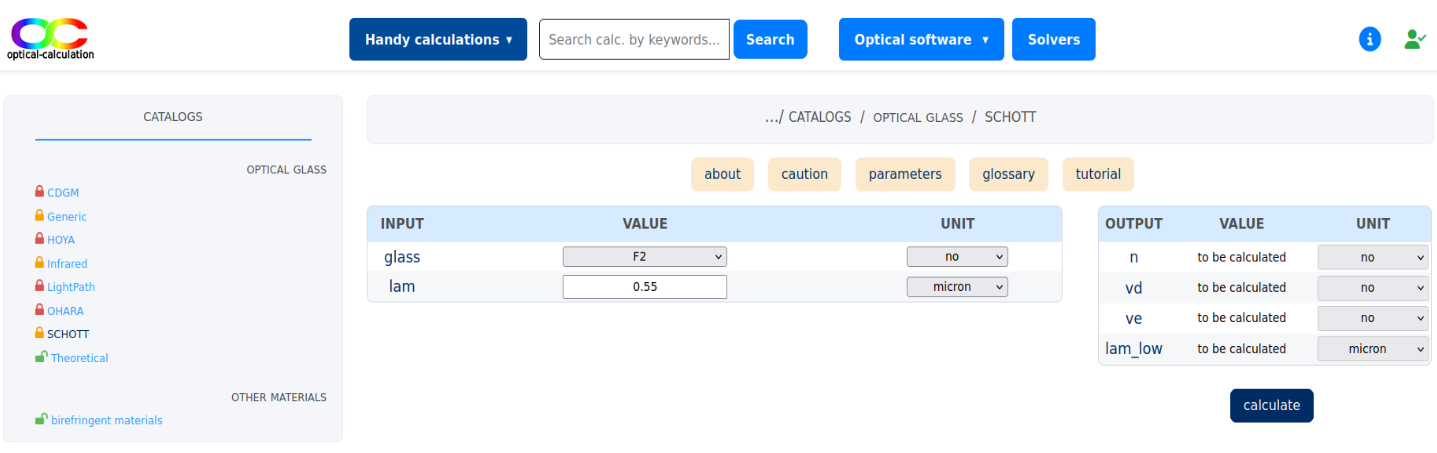Note that No other glass than the "theoretical" one can be used without logging. The "generic" catalog is available with a "student" license. All other catalogs can only be used with a "premium" license.

Entering a glass reference as a refraction index

In some pages, both refraction index and wavelength are input parameters. If entering a glass reference in the refraction index value cell instead of a number, the refraction index used in the calculation is the one of the reference at the entered wavelength.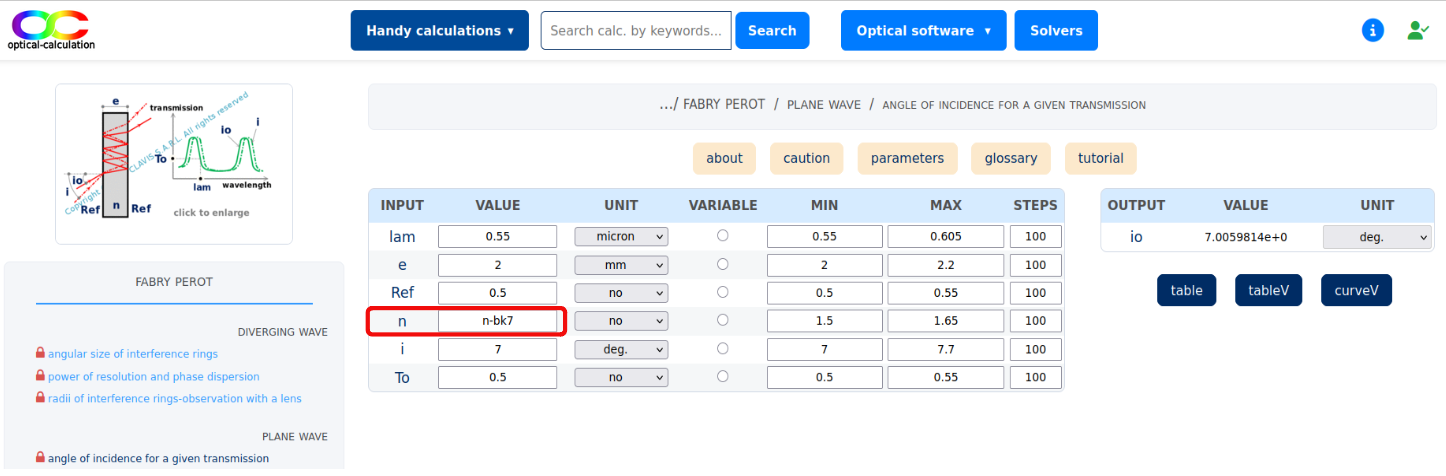In some other pages, the wavelength is not an input. when entering a glass reference in the refraction index cell, the refraction index used in the calculation is the one at a wavelength by default displayed on the top of the input tables when processing the calculation.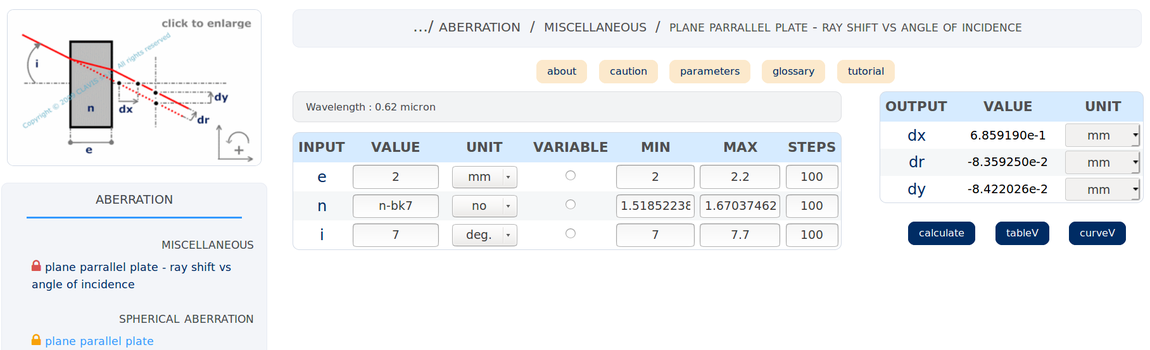Initially, the default wavelength is 0.55 microns. However, it can be changed by clicking on the wavelength page and enterring a new value in the "lam" cell. When the wavelength is an input parameter of the computation page, the enterred wavelength is also registered as the new default wavelength that can then be used in other calculations.

In the wavelength page, two other parameters can be changed: "lam_min" and "lam_max" which are respectively the minimum and maximum wavelengths used in the calculations related to chromatism.

Note that It is necessary that "lam_min" < "lam" < "lam_max", otherwise an error message is displayed.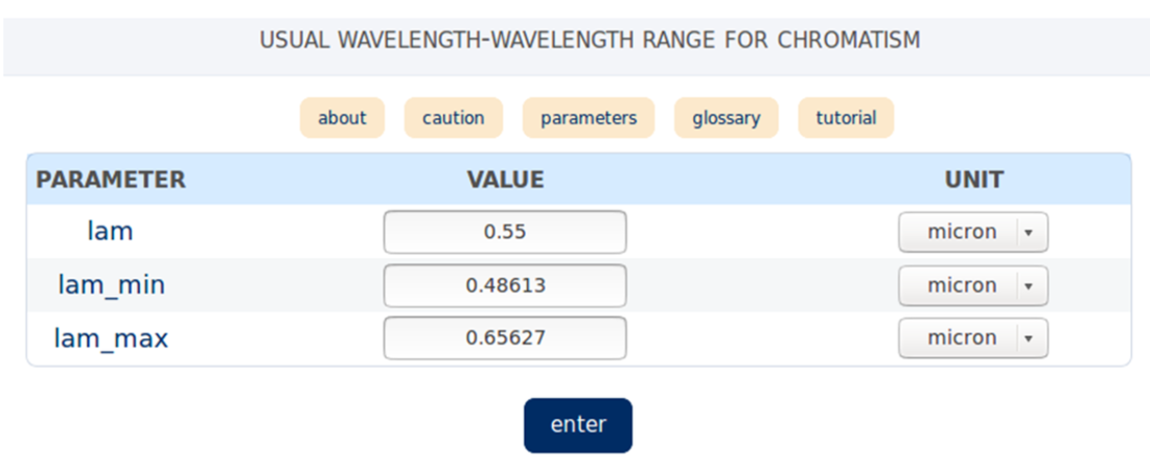For chromatism calculations, instead of enterring the refraction indexes of a medium corresponding to the smallest and the largest wavelengths of the considered spectrum, the user can also enter a glass reference from the catalogs defined in the catalogs link. The refraction indexes used at the smallest and largest wavelengths will be respectively those of the chosen reference respectively at the minimum and maximum default wavelengths which are automatically set at 0.48613 microns and 0.65627 microns but can be changed at the users convenience. These wavelengths are displayed on top of the input table once the calculation is processed.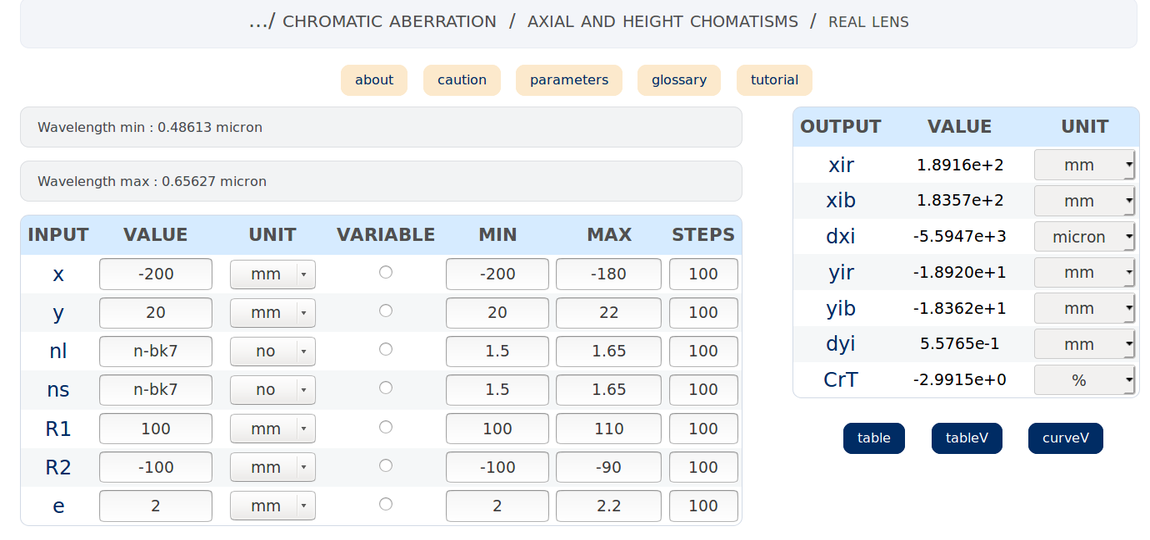When the constringence is an input, it can also either be a number or a glass reference. If it is defined by a glass referece, the calculation is processed with the selected glass constringence.